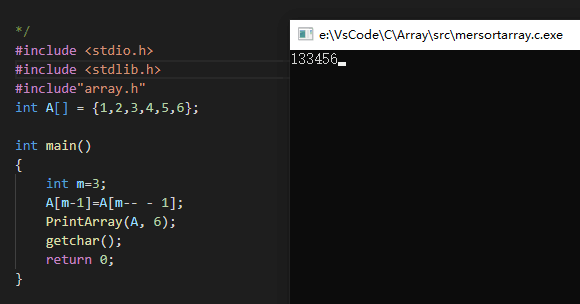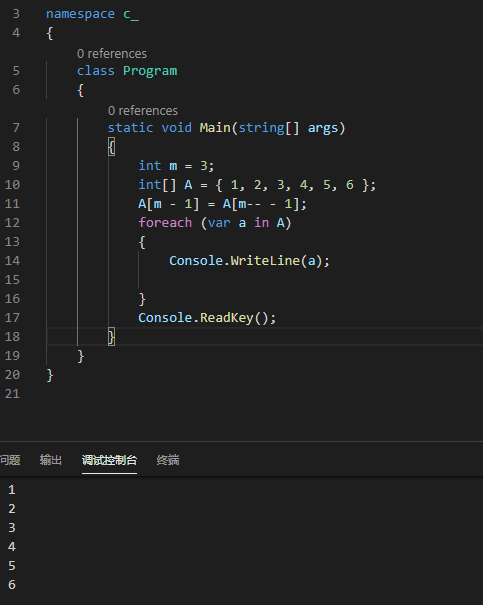### 简介

c语言：C#：void merge(int* A, int ASize, int m, int* B, int BSize, int n)//C语言
{

while (m > 0 && n > 0) {
// 对比选出较大的数放在 m + n - 1 的位置，并将选出此数的指针向前移动
A[m + n - 1] = A[m - 1] > B[n - 1] ? A[m-- - 1] : B[n-- - 1];
}
// 剩下的数都比已经遍历过的数小
// 如果 m 不为 0，则 A 没遍历完，都已经在 A 中不用再管
// 如果 n 不为 0，则 B 没遍历完，直接全移到 A 中相同的位置
while (n > 0) {
A[n - 1] = B[n - 1];
n--;
}
}

class Solution {
public void merge(int[] A, int m, int[] B, int n) //java语言
{
// 先确保将其中一个数组中的数字遍历完
while (m > 0 && n > 0) {
// 对比选出较大的数放在 m + n - 1 的位置，并将选出此数的指针向前移动
A[m + n - 1] = A[m - 1] > B[n - 1] ? A[m-- - 1] : B[n-- - 1];
}
// 剩下的数都比已经遍历过的数小
// 如果 m 不为 0，则 A 没遍历完，都已经在 A 中不用再管
// 如果 n 不为 0，则 B 没遍历完，直接全移到 A 中相同的位置
while (n > 0) {
A[n - 1] = B[n - 1];
n--;
}
}
}



Undefined Behavior.